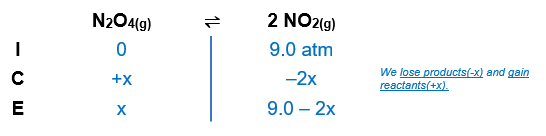# Problem: At a particular temperature, Kp = 0.25 for the reaction N2O4(g) ⇌ 2NO2(g)b. A flask containing only NO2 at an initial pressure of 9.0 atm is allowed to reach equilibrium. Calculate the equilibrium partial pressures of the gases.

###### FREE Expert Solution

N2O4(g) ⇌ 2NO2(g)              Kp = 0.25

Step 1: Construct an ICE chart for the equilibrium reaction.Step 2: Calculate the change (x) in the reaction using the equilibrium constant.

N2O4(g) ⇌ 2NO2(g)              Kp = 0.25

$\overline{){\mathbf{K}}{\mathbf{=}}\frac{\mathbf{products}}{\mathbf{reactants}}}\phantom{\rule{0ex}{0ex}}\mathbf{K}\mathbf{=}\frac{{\mathbf{\left[}{\mathbf{NO}}_{\mathbf{2}}\mathbf{\right]}}^{\mathbf{2}}}{\mathbf{\left[}{\mathbf{N}}_{\mathbf{2}}{\mathbf{O}}_{\mathbf{4}}\mathbf{\right]}}\phantom{\rule{0ex}{0ex}}\mathbf{0}\mathbf{.}\mathbf{25}\mathbf{=}\frac{{\mathbf{\left(}\mathbf{9}\mathbf{.}\mathbf{0}\mathbf{-}\mathbf{2}\mathbf{x}\mathbf{\right)}}^{\mathbf{2}}}{\mathbf{x}}\phantom{\rule{0ex}{0ex}}\mathbf{0}\mathbf{.}\mathbf{25}\mathbf{x}\mathbf{=}\mathbf{81}\mathbf{-}\mathbf{36}\mathbf{x}\mathbf{+}\mathbf{4}{\mathbf{x}}^{\mathbf{2}}\phantom{\rule{0ex}{0ex}}\overline{)\mathbf{0}\mathbf{=}\mathbf{4}{\mathbf{x}}^{\mathbf{2}}\mathbf{-}\mathbf{36}\mathbf{.}\mathbf{25}\mathbf{x}\mathbf{+}\mathbf{81}}$

Now, we will use the quadratic formula to solve for x. The quadratic formula is:

99% (53 ratings)###### Problem Details

At a particular temperature, Kp = 0.25 for the reaction

N2O4(g) ⇌ 2NO2(g)

b. A flask containing only NO2 at an initial pressure of 9.0 atm is allowed to reach equilibrium. Calculate the equilibrium partial pressures of the gases.

Frequently Asked Questions

What scientific concept do you need to know in order to solve this problem?

Our tutors have indicated that to solve this problem you will need to apply the ICE Chart concept. You can view video lessons to learn ICE Chart. Or if you need more ICE Chart practice, you can also practice ICE Chart practice problems.

What professor is this problem relevant for?

Based on our data, we think this problem is relevant for Professor Studer's class at South Texas College.

What textbook is this problem found in?

Our data indicates that this problem or a close variation was asked in Chemistry: An Atoms First Approach - Zumdahl Atoms 1st 2nd Edition. You can also practice Chemistry: An Atoms First Approach - Zumdahl Atoms 1st 2nd Edition practice problems.